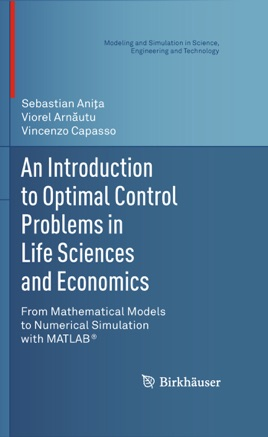• USD 64.99

## Descripción de editorial

Combining two important and growing areas of applied mathematics—control theory and modeling—this textbook introduces and builds on methods for simulating and tackling problems in a variety of applied sciences. Control theory has moved from primarily being used in engineering to an important theoretical component for optimal strategies in other sciences, such as therapies in medicine or policy in economics. Applied to mathematical models, control theory has the power to change the way we view biological and financial systems, taking us a step closer to solving concrete problems that arise out of these systems.

Emphasizing "learning by doing," the authors focus on examples and applications to real-world problems, stressing concepts and minimizing technicalities. An elementary presentation of advanced concepts from the mathematical theory of optimal control is provided, giving readers the tools to solve significant and realistic problems. Proofs are also given whenever they may serve as a guide to the introduction of new concepts. This approach not only fosters an understanding of how control theory can open up modeling in areas such as the life sciences, medicine, and economics, but also guides readers from applications to new, independent research.

Key features include:

* An introduction to the main tools of MATLAB®, as well as programs that move from relatively simple ODE applications to more complex PDE models;

* Numerous applications to a wide range of subjects, including HIV and insulin treatments, population dynamics, and stock management;

* Exploration of cutting-edge topics in later chapters, such as optimal harvesting and optimal control of diffusive models, designed to stimulate further research and theses projects;

* Exercises in each chapter, allowing students a chance to work with MATLAB and achieve a better grasp of the applications;